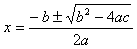The Formula

Unlike some of the other techniques discussed, the quadratic formula is a way to solve any quadratic equation of the form y = ax2 + bx + c. Substituting the values of a, b, and c into the equation below and simplifying will result in the solutions of the equation.

The Quadratic Formula is:.

To see how the formula can be derived by completing the square of y = ax2 + bx + c, select the link below: